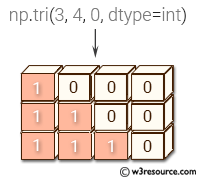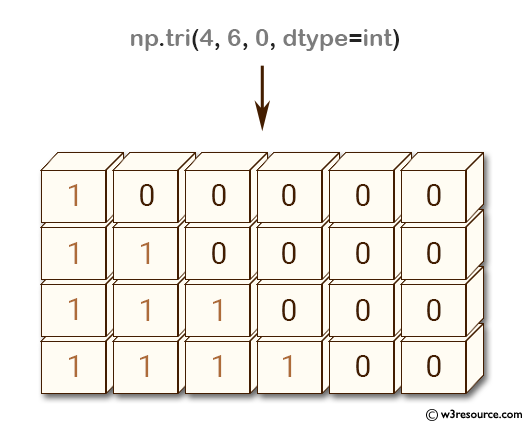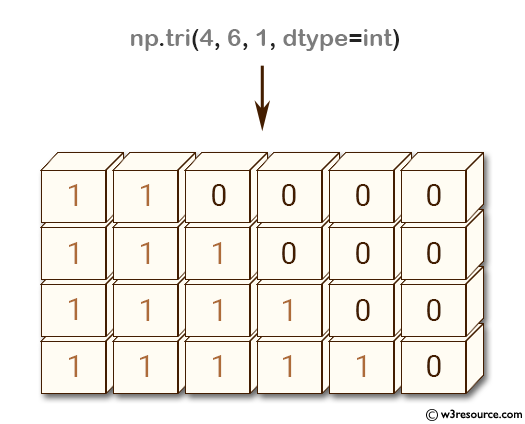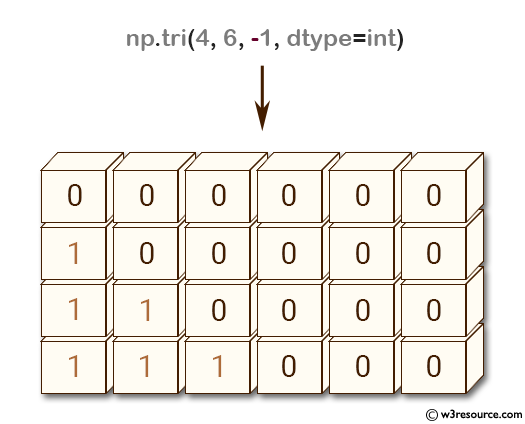# NumPy array creation: tri() function

## numpy.tri() function

The tri() function is used to get an array with ones at and below the given diagonal and zeros elsewhere.

Syntax:

`numpy.tri(N, M=None, k=0, dtype=<class 'float'>)`Version: 1.15.0

Parameter:

Name Description Required /
Optional
N Number of rows in the array. int
M Number of columns in the array. By default, M is taken equal to N. optional
k The sub-diagonal at and below which the array is filled. k = 0 is the main diagonal, while k < 0 is below it, and k > 0 is above. The default is 0. optional
dtype Data type of the returned array. The default is float. optional

Return value:

tri : ndarray of shape (N, M) - Array with its lower triangle filled with ones and zero elsewhere; in other words T[i,j] == 1 for i <= j + k, 0 otherwise.

Example-1: NumPy.tri() function main diagonal

``````>>> import numpy as np
>>> np.tri(4, 6, 0, dtype=int)
array([[1, 0, 0, 0, 0, 0],
[1, 1, 0, 0, 0, 0],
[1, 1, 1, 0, 0, 0],
[1, 1, 1, 1, 0, 0]])
``````

Pictorial Presentation:Example-2: NumPy.tri() function above main diagonal

``````>>> import numpy as np
>>> np.tri(4, 6, 1, dtype=int)
array([[1, 1, 0, 0, 0, 0],
[1, 1, 1, 0, 0, 0],
[1, 1, 1, 1, 0, 0],
[1, 1, 1, 1, 1, 0]])
``````

Pictorial Presentation:Example-3: NumPy.tri() function below main diagonal

``````>>> import numpy as np
>>> np.tri(4, 6, -1, dtype=int)
array([[0, 0, 0, 0, 0, 0],
[1, 0, 0, 0, 0, 0],
[1, 1, 0, 0, 0, 0],
[1, 1, 1, 0, 0, 0]])
``````

Pictorial Presentation:Python - NumPy Code Editor:

Previous: diagflat()
Next: tril()

﻿